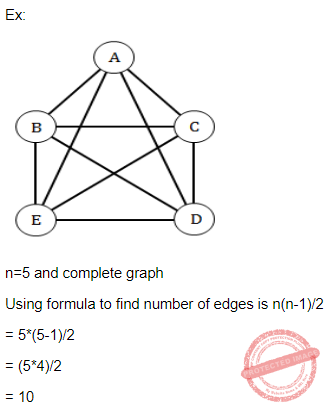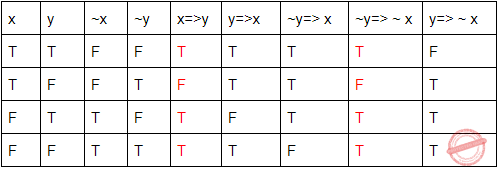## UGC NET CS 2007-Dec-Paper-2

 Question 1
A box contains six red balls and four green balls. Four balls are selected at random from the box. What is the probability that two of the selected balls are red and two are green ?
 A 3/7 B 4/7 C 5/7 D 6/7
Question 1 Explanation:Question 2
The number of edges in a complete graph with ‘n’ vertices is equal to :
 A n(n-1) B n(n-1)/2 C n2 D 2n-1
Question 2 Explanation:
Complete graph is an undirected graph in which each vertex is connected to every vertex, other than itself. If there are ‘n’ vertices then total edges in a complete graph is n(n-1)/2.Question 3
A context free grammar is :
 A type 0 B type 1 C type 2 D type 3
Question 3 Explanation:Question 4
Let e : B^m →B^n is a group code. The minimum distance of ‘e’ is equal to :
 A the maximum weight of a non zero code word B the minimum weight of a non zero code word C m D n
 Question 5
Consider a Moore machine M whose digraph is :Then L(M), the language accepted by the machine M, is the set of all strings having :
 A two or more b’s. B three or more b’s. C two or more a’s. D three or more a’s.
Question 5 Explanation:
In the given state diagram it can be clearly noticed that the minimum string that is accepted by the moore machine M is "bb". So the language can contain 0 or more number of a's and 2 or more number of b's.
 Question 6
A WFF that is equivalent to the WFF x=>y is :
 A y=> x B ~ y=> x C ~ y=> ~ x D y=> ~ x
Question 6 Explanation:Question 7
Simplified form of Boolean expression xy+(~x)z+yz is :
 A xy+(~x)z. B (~x)y+(~x)z. C (~x)y+xz. D xy+xz.
Question 7 Explanation:Question 8
In order to build a MOD-18 counter, the minimum number of flip flops needed is equal to :
 A 18 B 9 C 5 D 4
Question 8 Explanation:
Mod value of a counter is the number of unique states.
Let K = Number of states =18.
Number of flip-flops is the smallest number which is greater than or equal to Log K ( base 2).
Log 18 = 4.1.
L=5>=4.1.
So number of flip-flops needed is 5.
 Question 9
The dual of the switching function F=x+yz is given by :
 A x+yz B x(y+z) C (~x)+(~y)(~z) D (~x)((~y)+(~z))
Question 9 Explanation:
The dual of switching function means it changes AND to OR and OR to AND.
given x+yz.
x.(y+z)
 Question 10
Amongst the logic families DTL, TTL, ECL and CMOS, the family with the least power dissipation is :
 A CMOS B DTL C TTL D ECL
Question 10 Explanation:
Emitter Coupled Logic (ECL):
The storage time is eliminated as the transistors are used in difference amplifier mode and are never driven into saturation.
1. Fastest among all logic families
2. Lowest propagation delay.
Complementary metal oxide semiconductor(CMOS):
The power dissipation is usually 10nW per gate depending upon the power supply voltage, output load etc.
1. Lowest power dissipation 2. Excellent noise immunity 3. High packing density 4. Wide range of supply voltage 5. Highest fan out among all logic families Transistor Transistor Logic:
→ TTL devices consume substantially more power than equivalent CMOS devices at rest, but power consumption does not increase with clock speed as rapidly as for CMOS devices.
 Question 11
What cannot replace ‘?’ in the following C-code to print all odd numbers less than 100 ?
for (i=1; ? ; i=i+2)
printf(“%d\n”,i);
 A i≤100 B i≤101 C i<100 D i<101
Question 11 Explanation:
The given constraint in question, we have to print odd numbers in less than 100.
So, the condition should not be greater than 100 but it is equal to 100 acceptable.
for (i=1; i<=100 ; i=i+2)
printf(“%d\n”,i);
 Question 12Which of the following piece of code will insert the node pointed to by q at the end of the list ?
 A for (p=list; p !=NULL; p=p →next); p=q; B for (p=list; p !=NULL; p=p →next); p →next=q; C for (p=list; p →next!=NULL; p=p→next); p=q; D for (p=list; p →next !=NULL; p=p →next); p →next=q;
Question 12 Explanation:
→ n order to add a new node to the end of the list, first we need to traverse the entire list.
→ To the traverse the list, we will take one pointer.
→ We will traverse the list till the end by moving the pointer to next node.
→ The pointer will point to the last node and from there the pointer next address will point to the new node.
Option D will provide the above steps.
Q
There are 12 questions to complete.

Register Now